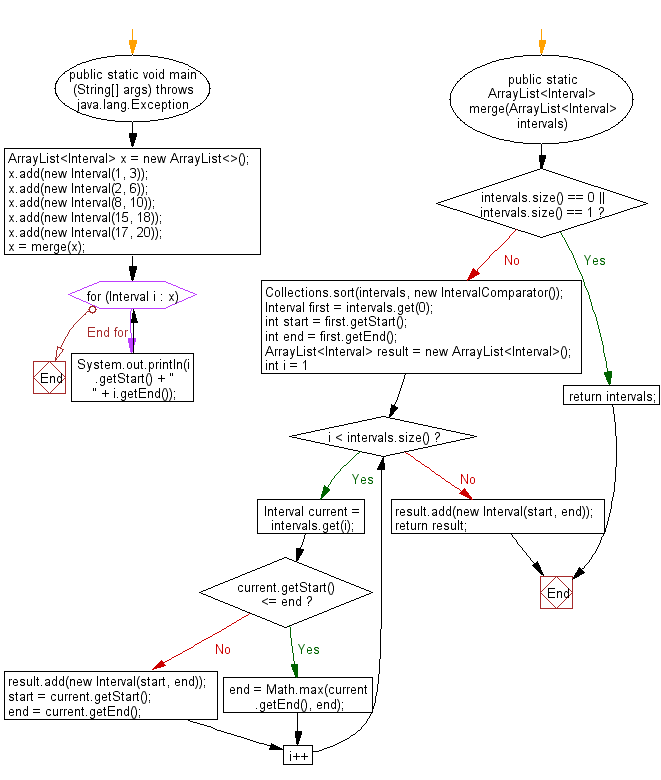﻿ Java: Merge all overlapping Intervals from a collection# Java Exercises: Merge all overlapping Intervals from a given a collection of intervals

## Java Basic: Exercise-140 with Solution

Write a Java program to merge all overlapping Intervals from a given a collection of intervals.

Sample Solution:

Java Code:

``````#// Source: https://bit.ly/2PjMfds
import java.util.*;

public class Solution
{
public static void main (String[] args) throws java.lang.Exception
{
ArrayList<Interval> x = new ArrayList<>();

x = merge(x);

for(Interval i : x)
{
System.out.println(i.getStart() + " " + i.getEnd());
}
}

public static ArrayList<Interval> merge(ArrayList<Interval> intervals) {

if(intervals.size() == 0 || intervals.size() == 1)
return intervals;

Collections.sort(intervals, new IntervalComparator());

Interval first = intervals.get(0);
int start = first.getStart();
int end = first.getEnd();

ArrayList<Interval> result = new ArrayList<Interval>();

for (int i = 1; i < intervals.size(); i++) {
Interval current = intervals.get(i);
if (current.getStart() <= end) {
end = Math.max(current.getEnd(), end);
} else {
start = current.getStart();
end = current.getEnd();
}
}

return result;
}
}

class Interval
{
private int start;
private int end;

Interval() {
start = 0;
end = 0;
}

Interval(int s, int e)
{
start = s;
end = e;
}

public int getStart() {
return start;
}

public int getEnd() {
return end;
}
}

class IntervalComparator implements Comparator<Interval>
{
public int compare(Interval i1, Interval i2)
{
return i1.getStart() - i2.getStart();
}
}
```
```

Sample Output:

```1 6
8 10
15 20
```

Flowchart:Java Code Editor:

What is the difficulty level of this exercise?

Test your Programming skills with w3resource's quiz.

﻿

## Java: Tips of the Day

countOccurrences

Counts the occurrences of a value in an array.

Use Arrays.stream().filter().count() to count total number of values that equals the specified value.

```public static long countOccurrences(int[] numbers, int value) {
return Arrays.stream(numbers)
.filter(number -> number == value)
.count();
}
```

Ref: https://bit.ly/3kCAgLb